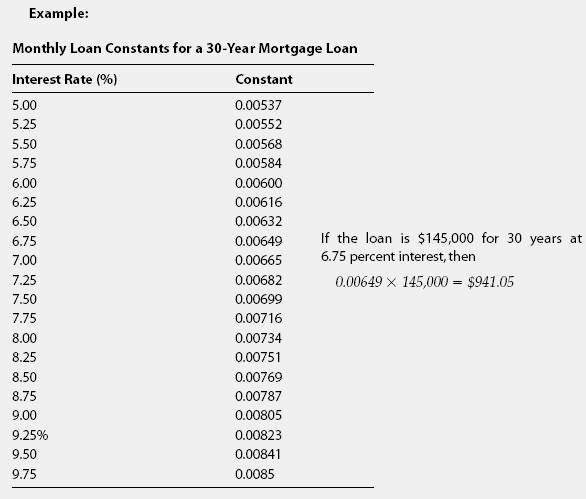# constant

(redirected from Binding constant)
Also found in: Dictionary, Thesaurus, Medical, Encyclopedia, Wikipedia.
Related to Binding constant: association constant

## constantThe annual payment required to pay the principal and interest due on a \$1 loan for a specified repayment term at a specified interest rate.Before the widespread availability of computers and extremely affordable financial calculators,it was common to use mortgage constant tables to calculate monthly payments. One would refer to the table for the proper loan term, such as 30 years, and then within that table find the loan constant for the anticipated interest rate. See the following example. The constant is the monthly payment on \$1. To find out your own monthly payment, you multiply the constant by the number of dollars in your loan, and the answer is what your monthly payments would be.

The Complete Real Estate Encyclopedia by Denise L. Evans, JD & O. William Evans, JD. Copyright © 2007 by The McGraw-Hill Companies, Inc.
References in periodicals archive ?
Plotting A0/A according to [Dt] A0/(A0-A) gives the binding constant K and the binding sites n according to the slope.
The relative absorbance I/[I.sub.0] of L (where [I.sub.0] and I represent the absorbance of L before and after the addition of an anion, respectively) upon the gradual addition of S[O.sub.4.sup.2-] gave the best fit to a 1:1 binding mode yielding a binding constant of 6.40 (in log K).
Binding constants of HBCD-RNA complexes were calculated as reported (37).
Aldrich-Wright, "Improved curve fitting procedures to determine equilibrium binding constants," Analyst, vol.
The linear fitting plots of log[([F.sub.0] - F)/F] versus log[Q] (Figure 5) from (3) furnished the values of intrinsic binding constant ([K.sub.b]) in the magnitude of 104-105, suggesting that the compounds bind strongly to DNA and the binding sites (n) were found to be 0.8-1.9 [+ or -] 0.15.
The binding mode, binding ratio, thermodynamic parameters, and binding constants were also obtained.
Table 1: Stern-Volmer constant ([K.sub.SV]), bimolecular quenching rate constant ([K.sub.q]), number of binding sites (n), binding constant ([K.sub.b]), and dissociation constant ([K.sub.d]) of several flavonoid-PDIA3 systems.
Unfortunately, due to the small changes registered on spectra as the titration occurs, these techniques are not appropriate to quantify the binding constant of the Au@tiopronin/DNA interaction.
The Quenching Mechanism and the Binding Constant. The binding constants of the static quenching were calculated according to the modified Stern-Volmer equation :
The apparent binding constant ([K.sub.app] = 1/[K.sub.d]) was calculated using 5) as described above and obtained as (1.81 [+ or -] 0.15) x [10.sup.4] [M.sup.-1] (Table 2).

Site: Follow: Share:
Open / Close